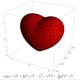# 惊人的答案：平均要取多少个(0,1)中的随机数才能让和超过1

数学常数最令人着迷的就是，它们常常出现在一些看似与之毫不相干的场合中。 随便取一个 0 到 1 之间的数，再加上另一个 0 到 1 之间的随机数，然后再加上一个 0 到 1 之间的随机数⋯⋯直到和超过 1 为止。一个有趣的问题：平均需要加多少次，才能让和超过 1 呢？答案是 e 次。为了证明这一点，让我们先来看一个更简单的问题：任取两个 0 到 1 之间的实数，它们的和小于 1 的概率有多大？容易想到，满足 x+y<1 的点 (x, y) 占据了正方形 (0, 1)×(0, 1) 的一半面积，因此这两个实数之和小于 1 的概率就是 1/2 。类似地，三个数之和小于 1 的概率则是 1/6 ，它是平面 x+y+z=1 在单位立方体中截得的一个三棱锥。这个 1/6 可以利用截面与底面的相似比关系，通过简单的积分求得：        ∫(0..1) (x^2)*1/2 dx = 1/6可以想到，四个 0 到 1 之间的随机数之和小于 1 的概率就等于四维立方体一角的“体积”，它的“底面”是一个体积为 1/6 的三维体，在第四维上对其进行积分便可得到其“体积”

∫(0..1) (x^3)*1/6 dx = 1/24

依此类推， n 个随机数之和不超过 1 的概率就是 1/n! ，反过来 n 个数之和大于 1 的概率就是 1 – 1/n! ，因此加到第 n 个数才刚好超过 1 的概率就是

(1 – 1/n!) – (1 – 1/(n-1)!) = (n-1)/n!

因此，要想让和超过 1 ，需要累加的期望次数为

∑(n=2..∞) n * (n-1)/n! = ∑(n=1..∞) n/n! = e

## 59 条评论

•question1663

BRILLIANT！

•voices

佩服!

•wecing

我靠，这个答案太悬疑了……

•Phil

您真的用 macbook 了?

•掌柜的马甲

e又是这它啊…

•Kane

突然发现源地址 http://www.mostlymaths.net 竟然被墙…

•Eagle_Fantasy

妙哉 无处不在的e

•白左

看见了mac疑似物

•白左

啊呀，所以说这是为什么呢，为什么宇宙中有一个数是大量规律的不变常数呢？
一个可能的答案是，为了减轻模拟我们这个宇宙的超级计算机的负荷，所有的积分常数和偏移常数都是一样的

超意识A:喂，给这个基准常数取一个数吧，整数？
（超意识B在单向加密软件中输入了他的名字，很快，一个长度为无限的无理数MD5i码就生成好了，前三位是2.71。超意识B随手将它复制进变量池最后一个空缺的位置，回车）

下一秒，宇宙诞生

•crush

不错！

•严酷的魔王

不错不错~

•shenpeng

刚刚用这个模型去求e的值，两次400000次重复试验试验，结果分别是2.71525和2.7154275，收敛好慢啊……

•青年

数学果然很美~

•DarkRaven

另有一个经典题目:
一串物品,每一个都有一个喜好度,一个一个给你看,你只能选择一次.
最佳方案是先看前n/e个,选出最大的,然后之后看到一个比这个最大值大的就选.
额,怎么证明呢?

•Melkor

算法导论里讲到了这个问题，随机算法

•DarkRaven

@白左:
无论在什么宇宙,只要数学系统相同,结果就是e,而数学是人类的创造!

•sexla

laiguangguangle，实在看不懂

•biohu

很神奇…

•剑影若兰

严格来说应该是满足[0,1]均匀分布的随机数吧。。。

•cbkid

博主也用mac了？linux没人了吗？

•ncmooc

这个答案令人深思。
惊现mac疑似物。

•猜的是3。。。还挺近的。。。一开始想随机取一个期望是0.5，所以大于两个的话基本就行。。。没想到这么复杂:D

•snaily

15L的问题也很有趣，求证！

•snaily

15L的问题有证明不？

•花荣

再引申一下，这些随机数加在一起，和是多少？
1.359?

•呼吸

看似毫不相干 我也这么想 幸会幸会

•Ming

一定要添加一条:
随机数是均匀分布在[0,1]上，否则结论不成立

我很喜欢你对于’n维立方体一角’体积的证明,很简单明了,得到1/n!的结果比我的笨方法好多了.
但是最后一步有点多余了,因为E(n|S(n)>1)=E(n|S(n)<1)=E(n|S(n)=1).

•wuzhengkai

归纳的很精妙！

•onlytest

这个是什么软件…

•Boleyn Su

首先，将题目转化为平均要取多少个1到n-1中的随机数才能让和超过n。显然当n趋于无穷大时，这两个问题是等效的。
然后，解：
设f(x)表示平均取多少次才能大于x.
显然 f(x)=0 x0来说,设之前最后一次取得了i,那么i在1到n-1之间,且取到任意一个数的概率为1/(n-1).
所以 f(x)=sum{(1+f(x-i))/(n-1);1<=i<=n-1}
=1+sum{f(x-i);1<=i<=n-1}/(n-1)
=1+sum{f(t);0<=t<=x-1}/(n-1)
令 F(x)=sum{f(i);0<=ioo) f(n)=(1+1/(n-1))^(n-1)*(1+1/(n-1))
=e*1
=e

•Boleyn Su

无语 XXXX为被和谐内容 这个HTML隔绝方式 也太奇特了吧
首先，将题目转化为平均要取多少个1到n-1中的随机数才能让和超过n。显然当n趋于无穷大时，这两个问题是等效的。
然后，解：
设f(x)表示平均取多少次才能大于x.
显然 f(x)=0 x<0
f(x)=1 x=0
对于x>0来说,设之前最后一次取得了i,那么i在1到n-1之间,且取到任意一个数的概率为1/(n-1).
所以 f(x)=sum{(1+f(x-i))/(n-1);1<=i<=n-1}
=1+sum{f(x-i);1<=i<=n-1}/(n-1)
=1+sum{f(t);0<=t<=x-1}/(n-1)
令 F(x)=sum{f(i);0<=i<=x}
则 f(x)=1+F(x-1)/(n-1) ……
所以 f(x-1)=1+F(x-2)/(n-1) ……
式-式得 f(x)-f(x-1)=(F(x-1)-F(x-2))/(n-1)
所以 (f(x)-f(x-1))*(n-1)=f(x-1)
所以 f(x)=f(x-1)*n/(n-1)
=(n/(n-1))^x*f(0)
=(n/(n-1))^x
所以 f(n)=(n/(n-1))^n
lim(n->+oo) f(n)=(1+1/(n-1))^(n-1)*(1+1/(n-1))
=e*1
=e

•math

加到第 n 个数才刚好超过 1 的概率就是

(1 – 1/n!) – (1 – 1/(n-1)!) = (n-1)/n!
这步怎么感觉不对啊,减是啥意思

•加力球

对于任意离散的随机变量N:
P{N = n} = P{N <= n} – P{N <= n – 1} 明白了吧

•sugarzh

关于15L的问题，魔方俱乐部里面有人讨论过这个

结论在主贴，证明在14L

•sugarzh

关于15L的问题，中国魔方俱乐部有人讨论过

结论在14L有证明

•sugarzh

– – 不小心风怒了。。。第一次卡了，刷新也刷不出来

•Nice Guy

从0到1取一个数小于1的概率也是1吗？！

•Nice Guy

n维空间与n-1维空间的运算这样不对吧

•aLex

15L本来是选秘书的题吧，大学概率书上有，很经典那，选老婆是不是也适用呀哈哈

•paramecium

昨天刚学了*中心极限定理*手痒想试试其威力的工科男解法：

对每一个随机数字xi服从均匀分布U(0,1)，均值E(xi)=1/2，方差D(xi)=1/12，令X=∑(i=1..n)xi，由中心极限定理，Zn = (X-∑E(xi)) / √∑D(xi)服从标准正态分布N(0,1)，P{X>1} = P{Zn>(1-n/2)/√(n/12)} = Φ((1-n/2)/√(n/12))。令(1-n/2)/√(n/12) (7+√33)/2=6.372，即平均*最少*要取6.372个随机数，X=Σxi>1，置信度超过99.87%(=99.74%+0.13%)。

•paramecium

发现少了字，再发一遍。

对每一个随机数字xi服从均匀分布U(0,1)，均值E(xi)=1/2，方差D(xi)=1/12，令X=∑(i=1..n)xi，由中心极限定理，Zn = (X-∑E(xi)) / √∑D(xi)服从标准正态分布N(0,1)，P{X>1} = P{Zn>(1-n/2)/√(n/12)} = Φ((1-n/2)/√(n/12))。令(1-n/2)/√(n/12)[小于号]-3σ=-3，解得n[大于号](7+√33)/2=6.372，即平均*最少*要取6.372个随机数，X=Σxi>1，置信度超过99.87%(=99.74%+0.13%)。

“尖括号”里面的字被吃掉了，强大的html过滤…

•paramecium

还有，31L的问题与原问题并不等价，最多只能说两个问题的答案刚好相等。M牛的问题是(0,1)范围内的实数集(不可数集)，而{1/n,2/n..1}是(0,1)范围内的有理数集的一个子集(可数集)，不可数集和可数集明显是不能一一对应的，31L的解法把所有的无理数都忽略掉了(比如√2/2)。

•yh

15L那个问题若干年前的mm群光棍节模拟赛里有。。

•ZWJ

如果n个数地和大于一常数K，那n的期望和K是什么函数关系呢？好像是分段的。用体积法可以证明K<1时，n=e^K。1<K<2时，n=e^K-(K-1)*e^(K-1)。后面的维数太高，几何直观出不来了。跪求高人啊！

•yang_bigarm

这个解法太高明了，我以前做过这个问题，先求均匀分布之和的表达式f[x]（这一步就巨复杂了），然后再算f[x]从0到1积分(又是一堆巨麻烦的积分)，可以得到相同的答案。

•mmaa01

我曾经考虑过一个问题：
一个数4，可以分解为4个相同的数之和吗？
一个数5，可以分解为5个相同的数之和吗？
一个数6，可以分解为6个相同的数之和吗？
结果发现都是1.
同样又想
一个数4，可以分解为4个相同的数之和吗？
一个数5，可以分解为5个相同的数之和吗？
一个数6，可以分解为6个相同的数之和吗？
答案是可以。但是每个分解都不一样。分别是4的4次根、5的5次根、6的6次根。大小不一样。
自然联想到哪个数的分解是最大的呢，结果是3的分解最大。
那么一个实数n可不可以考虑它是n（个）相等的数之乘积呢。就是n的1/n次方。
发现在实数域里面，通过求导可以得到，最大的那个分解的数是e。
用上面的语言描述就是e可以分解为e（个）相同的数之积。这个数是所有实数中按这个规律分解得到的最大的一个数1.4446678610097661336583391085964。（用xp自带的计算器算了一下，里面没有e，自己倒腾了几下不知道对不对）

•orbea jersey

这个理念不错 我很喜欢！

•AirLee

另一个问题：
定义运算Ranint（1,x)，x∈N，x＞1，是从1~x中随机取一个整数，设Xn+1=Ranint(1,Xn),X0=m时，若Xn=1，则期望E（n）=Hm≈ln（m),Hm为调和级数.
拓展：1、运算改为Ran(0,x),x∈R:从（0，x）中随机取一个实数，仿上迭代过程，当Xn＜1时结束，E（n）=？
2、运算改为Ran（0，kx）,k>0.k>e时，Xn有增加的趋势，k<e,Xn有减少的趋势，猜想E（Xn/X0)≈（k/e)^n,n趋向于正无穷.

两个拓展我都没搞出来，我觉得跟这个问题有渊源，求大牛解答.

•gml777

不错，不过看了一下博主都是 写概率方面的，其实数学除了概率还有很多很多

•cervelo

首先，将题目转化为平均要取多少个1到n-1中的随机数才能让和超过n。显然当n趋于无穷大时，这两个问题是等效的。

•lipan

31楼的答案跳跃的有点儿快。

•qirenrui

这个真神了……有没有本质些的解释

•ruiyang

厉害！！对于四维以上的，如四维 ∫(0..1) (x^3)*1/6 dx = 1/24，积分表达式不太理解。。。

•加力球

二维中面积比等于相似比平方，三维中体积比等于相似比立方，依此类推三维中面积比等于相似比立方, 可以类似推广到N维中的”体积”比等于相似比N次方
N维中的”锥体”是底面为一个N-1维图形, 并且在”高度”x处截得图行与底面相似且相似比为 (1-x) (假设高为1) 所以体积(也就是概率)
Pn = ∫(0,1)(1-x)^(n-1)P(n-1)dx, 得递推公式Pn = P(n-1) / n, 又有初值P1 = 1所以Pn = 1/n!